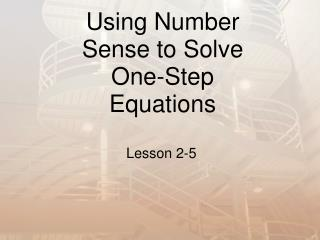# Using Number Sense to Solve One-Step Equations - PowerPoint PPT PresentationDownload PresentationUsing Number Sense to Solve One-Step Equations

Using Number Sense to Solve One-Step EquationsDownload Presentation## Using Number Sense to Solve One-Step Equations

- - - - - - - - - - - - - - - - - - - - - - - - - - - E N D - - - - - - - - - - - - - - - - - - - - - - - - - - -
##### Presentation Transcript

1. Vocabulary An equation is a mathematical sentence that has an equal sign. An open sentence is an equation with one or more variables in it. A solution is a value that can be substituted for a variable to make the equation true. Example: In the equation y + 3 = 8, the solution is 5.

2. Using Estimation • Use mental math or estimating to determine the solutions to the equations. • The same number properties apply to algebraic equations.

3. Properties Identity Properties: a + 0 = a1a = a Commutative Properties: a + b = b + aab = ba Associative Properties: a + (b + c) = (a + b) + ca(bc) = (ab)c

4. Example: 4x=32 Think: What times 4 equals 32? Solution: x = 8 Check the original equation: 4x=32 4(8)=32 32=32

5. Example: Estimatep ÷ 4 = 7.97 7.97 is close to 8. So think: What divided by 4 equals 8? If we work backwards, we know that 8 x 4 = 32. Therefore, p is about equal to 32.

6. Is the given number a solution to the equation? 6n = 17; 3 Try the value out and see if it works. 6n = 17 6(3) = 17 18 = 17 This is not true, so 3 is not a solution.

7. Homework Time